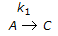# Chemical Engineering - Chemical Reaction Engineering

### Exercise :: Chemical Reaction Engineering - Section 2

16.

For a vapour phase catalytic reaction (A + B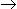P) which follows the Ridel mechanism and the reaction step is rate controlling, the rate of reaction is given by (reaction rate is irreversible, product also absorbs).

 A.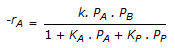B.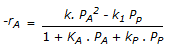C.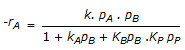D.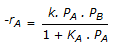Explanation:

No answer description available for this question. Let us discuss.

17.

If a solid-gas non-catalytic reaction occurs at very high temperature, the rate controlling step is the __________ diffusion.

 A. film B. ash layer C. pore D. none of these

Explanation:

No answer description available for this question. Let us discuss.

18.

The units of frequency factor in Arhenious equation

 A. are the same as those of the rate constant. B. depend on the order of the reaction. C. depend on temperature, pressure etc. of the reaction. D. are cycles per unit time.

Explanation:

No answer description available for this question. Let us discuss.

19.

Which of the following is not a chemical step in a fluid solid catalytic reaction ?

 A. Surface chemical reaction B. Adsorption C. Desorption D. None of these

Explanation:

No answer description available for this question. Let us discuss.

20.

For a series of reactions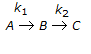having k1 << k2, the reaction system can be approximated as

 A.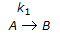B.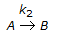C.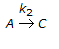D.